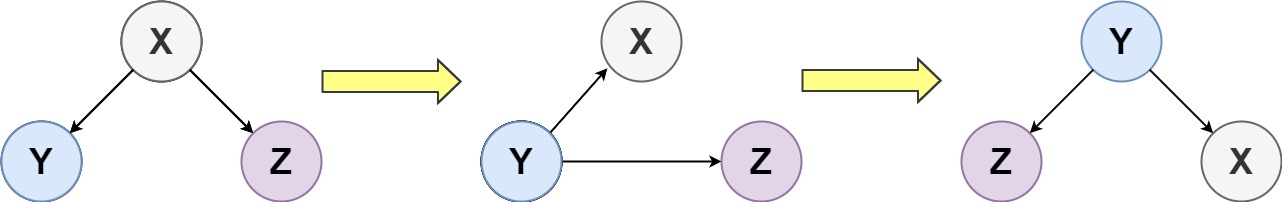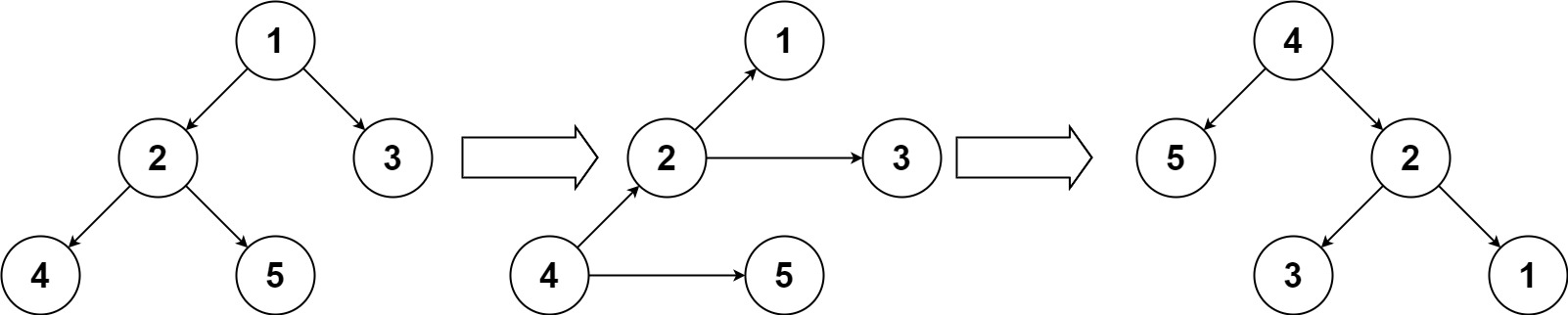## 156. Binary Tree Upside Down

Given the `root` of a binary tree, turn the tree upside down and return the new root.

You can turn a binary tree upside down with the following steps:

1. The original left child becomes the new root.
2. The original root becomes the new right child.
3. The original right child becomes the new left child.The mentioned steps are done level by level, it is guaranteed that every node in the given tree has either 0 or 2 children.

Example 1:```Input: root = [1,2,3,4,5]
Output: [4,5,2,null,null,3,1]
```

Example 2:

```Input: root = []
Output: []
```

Example 3:

```Input: root = 
Output: 
```

Constraints:

• The number of nodes in the tree will be in the range `[0, 10]`.
• `1 <= Node.val <= 10`
• `Every node has either 0 or 2 children.`

## Rust Solution

``````struct Solution;
use rustgym_util::*;

trait Preorder {
}

if let Some(node) = self {
let left_tree = node.borrow_mut().left.take();
let right_leaf = node.borrow_mut().right.take();
node.borrow_mut().left = left;
node.borrow_mut().right = right;
left_tree.preorder(right_leaf, Some(node))
} else {
right
}
}
}

impl Solution {